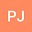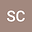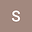Composite Higgs Model parameter determination at the FCC-ee
•••• Patrick Janot,
• stefania de curtis,
• s.moretti
Patrick Janot
European Organization for Nuclear Research (CERN), CERN Pilot
Author Profilestefania de curtis
Author Profile## Abstract

We assess the scope of a Future Circular Collider operating in $$e^{+}e^{-}$$ mode (FCC-ee) in accessing the parameter space of the 4-Dimensional Composite Higgs Model (4DCHM), which represents a realistic implementation of Electro-Weak Symmetry Breaking (EWSB) dynamics triggered by a pseudo-Nambu-Goldstone Boson (pNGB) emerging from the breaking of a global symmetry group $$SO(5)\to SO(4)$$ describing new strong interactions eventually resposible for Higgs compositeness. In fact, in such a framework, other composite states exist, like heavy spin-1 bosons ($$W^{\prime}$$s and $$Z^{\prime}$$s) and spin-1/2 fermions ($$b^{\prime}$$s and $$t^{\prime}$$s). Herein, we concentrate initially on the neutral gauge boson sector of this scenario, by attempting to extract the (modified) couplings of the Standard Model (SM) $$Z$$ state as well as the masses and quantum numbers of the additional $$Z^{\prime}$$ objects present in the 4DCHM. We establish the sensitivity of an FCC-ee to such parameters via the processes $$e^{+}e^{-}\to\mu^{+}\mu^{-}$$ and $$e^{+}e^{-}\to t\bar{t}$$ for a varieties of foreseen energies and luminosities, by exploiting both cross section and asymmetry observables. We finally combine the results obtained in the $$Z^{\prime}$$ sector of the 4DCHM with those emerging from foreseen Higgs measurements, as previously assessed, so as to delineate an analysis programme aimed at confirming or disproving once and for all the validity of the compositeness paradigm.